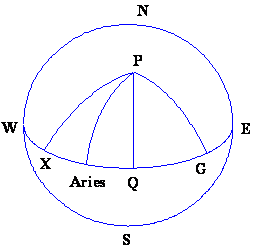Back to Nautical Solar Sys. Hour Angles PZX Triangles Dip Amplitude Day Ex Meridian Exercises Pole Star Naut. Almanac

Hour Angles

Since the Greenwich meridian rotates with the earth from west to east, whereas each hour circle remains linked with the almost stationary position of the respective body in the sky, the GHA’s of all celestial bodies increase as time progresses (approx. 15° per hour).

In contrast to stars, the GHA’s of sun, moon, and planets increase at slightly different (and variable) rates.

This is attributable to the revolution of the planets (including the earth) around the sun and to the revolution of the moon around the earth, resulting in additional apparent motions of these bodies in the sky.

It is sometimes useful to measure the angular distance between the hour circle of a celestial body and the hour circle of a reference point in the sky instead of the Greenwich meridian because the angle thus obtained is independent of the earth’s rotation.

The angular distance of a body (X) westward from the hour circle (upper branch) of the first point of Aries, measured from 0° through 360° is called sidereal hour angle, SHA.

The first point of Aries is the fictitious point in the sky where the sun passes through the plane of the earth’s equator in spring (vernal point). The GHA of a body is the sum of the SHA of the body and the GHA of the first point of Aries, GHA Aries:

(If the resulting GHA is greater than 360°, subtract 360°.)

GHA Aries, measured in time units (0-24h) instead of degrees, is called Greenwich Sidereal Time, GST:

The angular distance of a body measured in time units (0-24h) eastward from the hour circle of the first point of Aries is called right ascension, RADeclinations are not affected by the rotation of the earth.

The concept of the earth’s axial rotation causing change in the hour angle of bodies

Since the Greenwich meridian rotates with the earth from west to east, whereas each hour circle remains linked with the almost stationary position of the respective body in the sky, therefore the GHA’s of all celestial bodies increase as time progresses (approx. 15° per hour).

In contrast to stars, the GHA’s of sun, moon, and planets increase at slightly different (and variable) rates. This is due to the revolution of the planets (including the earth) around the sun and to the revolution of the moon around the earth, resulting in additional apparent motions of these bodies in the sky.

It is sometimes useful to measure the angular distance between the hour circle of a celestial body and the hour circle of a reference point in the sky instead of the Greenwich meridian because the angle thus obtained is independent of the earth’s rotation.

The angular distance of a body westward from the hour circle (upper branch) of the first point of Aries, measured from 0° through 360° is called siderial hour angle, SHA.

The first point of Aries is the fictitious point in the sky where the sun passes through the plane of the earth’s equator in spring (vernal point).

The GHA of a body is the sum of the SHA of the body and the GHA of the first point of Aries

‘Greenwich Hour Angle (GHA)’, ‘Local Hour Angle (LHA)’and longitude, and their relationship

The Greenwich hour angle, GHA, is the angular distance of a Geographical Position westward from the upper branch of the Greenwich meridian (0°), measured from 0° through 360°.

The declination, Dec, is the angular distance of Geographical Position from the plane of the equator, measured northward through +90° or southward through -90°.

GHA and Dec are geocentric coordinates (measured at the center of the earth).

The great circle going through the poles and Geographical Position is called hour circle.

The rate of change of GHA of the sun and Aries

Since the Greenwich meridian rotates with the earth from west to east, whereas each hour circle remains linked with the almost stationary position of the respective body in the sky, the GHA’s of all celestial bodies and the imaginary point of aries increase as time progresses (approx. 15° per hour).

In contrast to stars, the GHA’s of sun, moon, and planets increase at slightly different (and variable) rates. This is attributable to the revolution of the planets (including the earth) around the sun and to the revolution of the moon around the earth, resulting in additional apparent motions of these bodies in the sky.

It is sometimes useful to measure the angular distance between the hour circle of a celestial body and the hour circle of a reference point in the sky instead of the Greenwich meridian because the angle thus obtained is independent of the earth’s rotation. The angular distance of a body westward from the hour circle (upper branch) of the first point of Aries, measured from 0° through 360° is called siderial hour angle, SHA. The first point of Aries is the fictitious point in the sky where the sun passes through the plane of the earth’s equator in spring (vernal point). The GHA of a body is the sum of the SHA of the body and the GHA of the first point of Aries,

The geographical position of a body for any given GMT

Star

From the Almanac take down the GHA (Aries) for the GMT in question

Take down the SHA of the star

Add the two and GHA (Star) is obtained,

Take down the Declination of the Star.

Use the GHA (Star) as the Longitude and the Declination as the Latitude and plot on the chart; this would be the GP of the Star.

Sun

Take down the GHA (Sun) from the almanac and the Declination. Use the Declination as the Latitude and the GHA (Sun) as the longitude to plot on the chart.